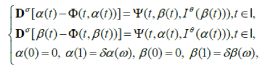# Matrix Science Mathematic (MSMK)

### Study of Nonlocal Boundary Value Problems of Non-Integer Order Hybrid Differential Equations

February 26, 2019 Posted by Nurul In MSMK

## ABSTRACT

### Study of Nonlocal Boundary Value Problems of Non-Integer Order Hybrid Differential Equations

Journal: Matrix Science Mathematic (MSMK)
Author: Samina, Kamal Shah and Rahmat Ali Khan

This is an open access article distributed under the Creative Commons Attribution License, which permits unrestricted use, distribution, and reproduction in any medium, provided the original work is properly citedWe study sufficient conditions for existence and uniqueness of solutions to boundary value problems (BVPs) for fractional hybrid differentialequations(FHDEs) of the formwhere I=[0,1],σ,θ ∈(1,2] and δ,ω∈(0,1) . We use hybrid fixed point theorem due to Dhage and develop adequateresults for existence of solutions to the proposed system of (FHDEs). We also provide a numerical problem to demonstrate our main results.
 Pages 21-24 Year 2017 Issue 1 Volume 1